# Overview

The regmed method performs regularized mediation analysis based on penalized structural equation modeling. The original version (version 1.x) on CRAN was for structural equations with multiple mediators between a single exposure variable and a single outcome variable. The penalized model implemented in the regmed functions is based on sparse group lasso in which the pair of parameters alpha and beta are considered a group; alpha is the effect of exposure on a mediator and beta is the effect of that mediator on the outcome. Version 2.x and later now include computationally efficient models that allow for multiple exposures, multiple mediators, and multiple outcomes. The penalized model for this multivariate situation, implemented in functions denoted mvregmed, uses a lasso (L1) type of penalty for all parameters: alpha linking exposures with mediators; beta linking mediators with outcomes; and delta linking exposures directly with outcomes.

The default analysis approach is to center and scale all x, mediator, and y variables, so each column of the x, mediator, and y variables has mean 0 and variance 1. This default approach is recommended because it places all variables on the same scale. If adjustment for extraneous covariates is needed, regression of any of the variables on covariates should be performed and the residuals from these regression models can be used as input variables to the methods of regmed or mvregmed. When standardized variables are used, the penalized structural equation models are modeling the correlations of variables.

## Simulated dataset

The simulated data set (named “medsim”) contains 100 subjects with 10 exposure “x” variables, 200 mediator “med” variables, and 2 outcome “y” variables.

data(medsim)

# regmed: Mediation model for 1 exposure, multiple mediators, and 1 outcome variable

This section focuses on the original version of regmed that handles a single exposure and a single outcome. The user-level functions for single outcome/exposure are:

• regmed.prefilter() pre-filters mediators if number of mediators is greater than the number of subjects
• regmed.grid() penalized model for grid of lambdas
• regmed.grid.bestfit() get best fit model from grid of fits
• regmed.fit() fit penalized model with specified lambda
• plot.regmed.grid() plot results of regmed.grid
• regmed.edges() create edges from a fit of a model for use in plotting and model fit by lavaan
• plot.regmed.edges() plot directed graph based on edges: exposure -> mediator -> outcome
• regmed.lavaan.model() setup model to estimate unpenalized parameters by lavaann sem function
• regmed.lavaan.dat() setup data to input to lavaann sem function
• summary.lavaan() summarize lavaan sem model fit

## Pre-filter mediators

If the number of mediators exceeds the sample size, model fitting can become unstable. To reduce the number of mediators to fit, the regmed.prefilter uses sure independence screening (Fan & Lv, 2008) to reduce the number of potential mediators. This is based on ranking marginal correlations and then selecting the highest ranked values such that the number of parameters is less than the sample size. Because mediation depends on the two correlations, $$cor(x,med)$$ and $$cor(med,y)$$ we rank the absolute values of their products, $$|cor(x, med) * cor(med, y)|$$, and choose the highest k ranked values to determine which potential mediators to include in penalized mediation models. If k is not specified, the default value of k is n/2, where n is the sample size, because each mediator results in two parameters alpha and beta.

To speed calculations solely for demonstration purposes, we choose k=10 mediators. Also, we select the first exposure variable and the first outcome variable to demonstrate methods for regmed. The function regmed.prefilter returns a list of x, mediator, and y, with each of these variables centered and scaled by default, and accounts for missing data by subsetting to subjects that are not missing any of the variables.

dat.filter <- regmed.prefilter(x[, 1], med, y[, 1], k = 10)
colnames(dat.filter$mediator)   "med.1" "med.2" "med.74" "med.88" "med.90" "med.99" "med.112"  "med.129" "med.133" "med.178" ## Grid of lambda penalties To fit a series of models over a grid of penalty “lambda” values, it is necessary to define a vector of lambda values. It is best to arrange this vector from largest to smallest lambda values to assure that the largest lambda (first value in the vector) results in no selected parameters (alpha, beta, delta), and the smallest lambda (last value in the vector) selects multiple parameters, and that a lambda between the largest and smallest results in a minimum Bayesian Information Criterion (BIC). Starting with a large lambda and then decreasing lambda values is best, because this provides a “warm start” for each subsequent fit, with final fitted parameter values for a specific lambda used as initial values for the next lambda value. The demonstration below extracts variables that have been processed by regmed.prefilter. ## Fit regmed for grid of lambda penalties lambda.grid <- seq(from = 0.4, to = 0.01, by = -0.05) x1 <- dat.filter$x
y1 <- dat.filter$y med <- dat.filter$mediator

fit.grid <- regmed.grid(x1, med, y1, lambda.grid, frac.lasso = 0.8)

The plot method for regmed.grid gives two figures that represent the size of the coefficients over the grid of lambdas, and the BIC by by the grid of lambdas.

print(fit.grid)
Call:
regmed.grid(x = x1, mediator = med, y = y1, lambda.vec = lambda.grid,
frac.lasso = 0.8)

lambda converge iter df      bic
1   0.40     TRUE  289  9 1048.155
2   0.35     TRUE    8  9 1041.148
3   0.30     TRUE    5 12 1047.839
4   0.25     TRUE   14 15 1053.422
5   0.20     TRUE    6 17 1053.775
6   0.15     TRUE   14 19 1055.445
7   0.10     TRUE   15 21 1059.531
8   0.05     TRUE    9 21 1059.378
plot.regmed.grid(fit.grid)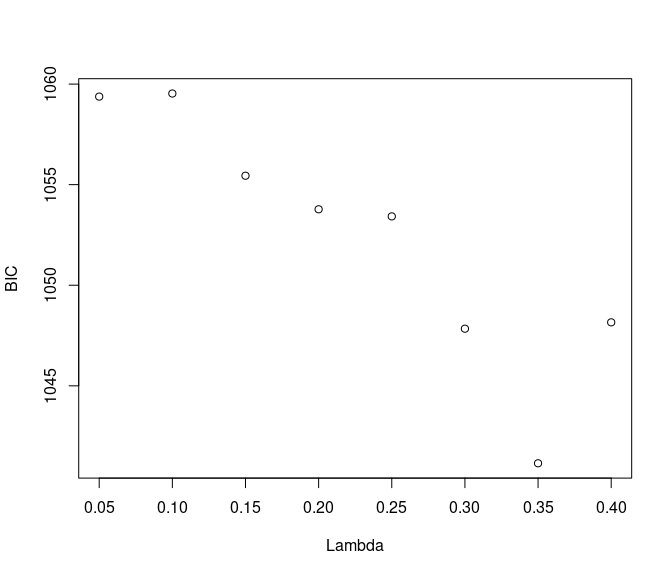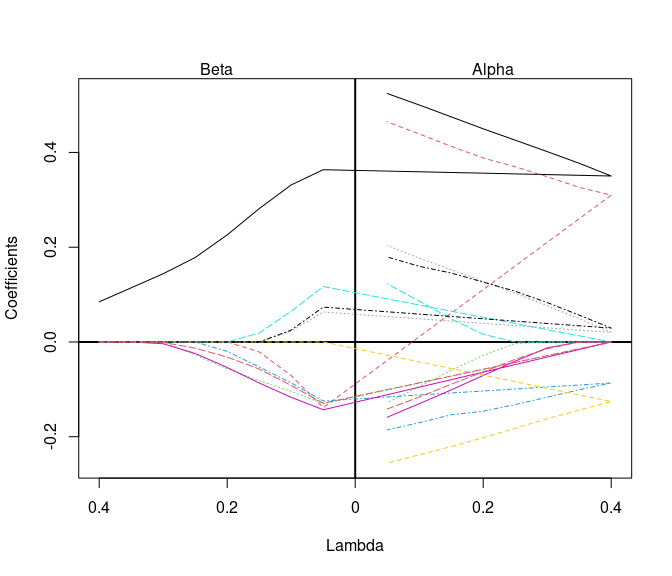## Select best model from grid by minimum BIC

fit.best <- regmed.grid.bestfit(fit.grid)
summary(fit.best)
Call:
NULL

Coefficients:
alpha      beta alpha*beta
med.1    0.37711665 0.1143248 0.04311379
med.2    0.32632288 0.0000000 0.00000000
med.74   0.00000000 0.0000000 0.00000000
med.88  -0.10086322 0.0000000 0.00000000
med.90   0.00000000 0.0000000 0.00000000
med.99   0.00000000 0.0000000 0.00000000
med.112 -0.14386830 0.0000000 0.00000000
med.129  0.04906179 0.0000000 0.00000000
med.133  0.05666569 0.0000000 0.00000000
med.178  0.00000000 0.0000000 0.00000000

sum of alpha*beta =  0.04311379
delta =  0.5050343
sum of delta + alpha*beta =  0.5481481
var(x) =  1
var(y) =  1.101601 

## Fit single regmed model with user-specified lambda

If user prefers to specify a lambda penalty and not search through a grid, the function regmed.fit is available.

fit.single <- regmed.fit(x1, med, y1, lambda = 0.3, frac.lasso = 0.8)
summary(fit.single)
Call:
regmed.fit(x = x1, mediator = med, y = y1, lambda = 0.3, frac.lasso = 0.8)

Coefficients:
alpha         beta   alpha*beta
med.1    0.40109014  0.126420020 5.070582e-02
med.2    0.35582882  0.000000000 0.000000e+00
med.74   0.00000000  0.000000000 0.000000e+00
med.88  -0.13906482  0.000000000 0.000000e+00
med.90   0.00000000  0.000000000 0.000000e+00
med.99  -0.01045770 -0.001413514 1.478211e-05
med.112 -0.17587944  0.000000000 0.000000e+00
med.129  0.07206755  0.000000000 0.000000e+00
med.133  0.07530145  0.000000000 0.000000e+00
med.178 -0.01914775  0.000000000 0.000000e+00

sum of alpha*beta =  0.05072061
delta =  0.5386689
sum of delta + alpha*beta =  0.5893895
var(x) =  1
var(y) =  1.116486 

## Plot directed graph of fit model

The demonstration below shows how to determine edges, where an edge is defined by vertex-1 pointing to vertex-2, and the vertices are the variables selected in a model. For example, if alpha and beta are both estimated to be non-zero, then x -> med and med -> y. The function regmed.edges has an option to choose how vertices and edges are selected. By type=“mediators”, vertices and edges are selected only if the product $$alpha * beta$$ is non-zero, because this is required for the definition of a mediator. By specifying type = “any”, all vertices and edges that represent non-zero parameters are selected. For example, if alpha is non-zero, and beta is zero, the edge x -> med is selected, but the edge med -> y is not selected. To determine if terms are zero, a threshold variable eps is used (see help for regmed.edges).

For fit.best, the summary above shows that only med.1 is selected as a mediator, because $$|alpha * beta| > eps$$. In this case, choosing type=“mediators” selects only med.1 to be included in edges.

In contrast, choosing type=“any” for fit.best includes more mediator variables with edges, even though they do not all meet the definition of a mediator.

edges.med <- regmed.edges(fit.best, type = "mediators")
plot.regmed.edges(edges.med)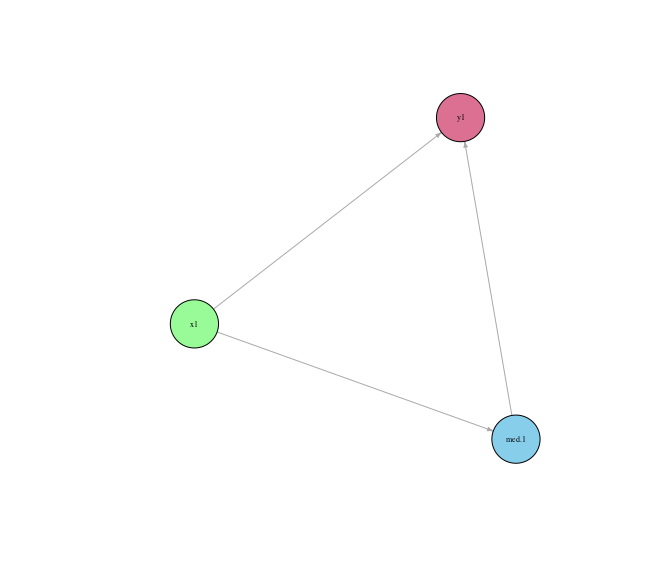edges.any <- regmed.edges(fit.best, type = "any")
plot.regmed.edges(edges.any)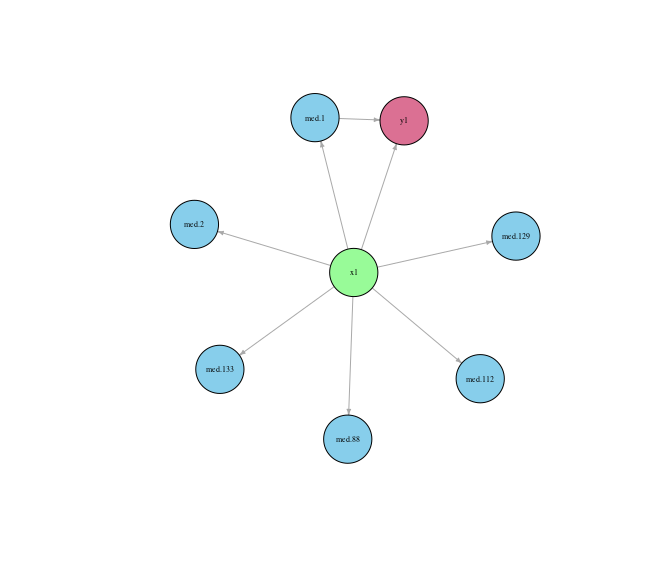## Lavaan sem: Estimate parameters of selected model without imposing penalty

Because penalized models can overly shrink parameter estimates towards zero, it can be beneficial to refit the selected model without penalties. The model without penalties is a standard structural equation model, which can be fit with the function sem from the package lavaan. To facilitate this step, the function regmed.lavaan.model uses the edges and fit of a model to create a text string that represents the model syntax for sem. This function abides by our assumption that the residual covariances between x and med, between x and y, and between med and y, are all zero. It also assumes that the covariances of the mediators, a matrix in the fit of a regmed object, are fixed in the sem model fitting.

The demonstration below shows how to set up the data set and models for sem. The function regmed.lavaan.dat assures that x, med, y, are centered and scaled and subset to subjects without any missing data.

dat <- regmed.lavaan.dat(x1, med, y1)

mod.best <- regmed.lavaan.model(edges.med, fit.best)

mod.any <- regmed.lavaan.model(edges.any, fit.best)

The demonstration below shows how to use lavaan:::sem() to fit specified models and view results

fit.lav.med <- lavaan:::sem(model = mod.best, data = dat)
summary.lavaan(fit.lav.med)
    lhs op   rhs   est    se     z pvalue ci.lower ci.upper
1 med.1  ~    x1 0.551 0.084 6.577  0.000    0.387    0.716
2    y1  ~ med.1 0.293 0.093 3.147  0.002    0.110    0.475
3    y1  ~    x1 0.415 0.093 4.445  0.000    0.232    0.598
4    y1 ~~    y1 0.602 0.085 7.071  0.000    0.435    0.769
fit.lav.any <- lavaan:::sem(model = mod.any, data = dat)
summary.lavaan(fit.lav.any)
      lhs op   rhs    est    se      z pvalue ci.lower ci.upper
1   med.1  ~    x1  0.551 0.084  6.577  0.000    0.387    0.716
2   med.2  ~    x1  0.489 0.088  5.576  0.000    0.317    0.661
3  med.88  ~    x1 -0.197 0.099 -2.002  0.045   -0.390   -0.004
4 med.112  ~    x1 -0.264 0.097 -2.723  0.006   -0.454   -0.074
5 med.129  ~    x1  0.232 0.098  2.369  0.018    0.040    0.423
6 med.133  ~    x1  0.200 0.098  2.032  0.042    0.007    0.393
7      y1  ~ med.1  0.293 0.093  3.147  0.002    0.110    0.475
8      y1  ~    x1  0.415 0.093  4.445  0.000    0.232    0.598
9      y1 ~~    y1  0.602 0.085  7.071  0.000    0.435    0.769

# mvregmed : Mediation model for multiple exposures, multiple mediators, and multiple outcome variables

New in version 2.0 is the multivariate version mvregmed, with the mv short for multivariate. The available user-level functions are listed as follows:

• mvregmed.grid() penalized model for grid of lambdas
• mvregmed.grid.bestfit() get best fit mvregmed model from grid of fits
• mvregmed.fit() fit penalized mvregmed model with specified lambda
• plot.mvregmed.grid() plot results of mvregmed.grid
• plot.mvregmed() plot results of a single mv regmed model fit, from either mvregmed.fit or mvregmed.grid.bestfit
• mvregmed.edges() create edges from a fit of a model for use in plotting and model fit by lavaan
• plot.mvregmed.edges() plot directed graph based on edges: exposure -> mediator -> outcome
• regmed.lavaan.model() setup model to estimate unpenalized parameters by lavaann sem function
• regmed.lavaan.dat() setup data to input to lavaann sem function
• summary.lavaan() summarize lavaan sem model fit, which applies to both regmed.fit and mvregmed.fit runs in the lavaan::sem, because the outputs are the same.

## Fit mvregmed for a grid for lambda penalties

With a specified lambda.grid, fit a grid of models over the multiple exposures and mediators. We see in the summary and the plot that a lambda around 0.10 provides the best model (lowest bic).

fit.grid <- mvregmed.grid(x, med, y, lambda.grid)
summary(fit.grid)
  lambda converge iter df df.alpha df.beta df.delta df.vary      bic
1   0.40     TRUE    1  3        0       0        0       3 1654.792
2   0.35     TRUE    1  3        0       0        0       3 1654.792
3   0.30     TRUE   41  6        1       1        1       3 1648.337
4   0.25     TRUE   26  8        2       2        1       3 1615.818
5   0.20     TRUE   21  8        2       2        1       3 1574.292
6   0.15     TRUE   18 14        4       5        2       3 1562.402
7   0.10     TRUE   14 24       10       7        4       3 1551.387
8   0.05     TRUE   24 48       21      14       10       3 1593.028
plot(fit.grid)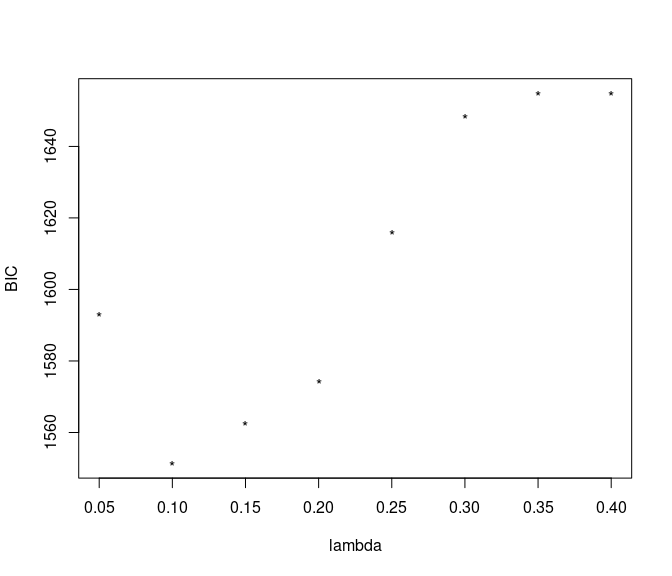## Select best model from grid based on minimum BIC

Choose best fit model from grid based on min BIC.

mvfit.best <- mvregmed.grid.bestfit(fit.grid)

Instead of examining all contents of a model, use summary to view non-zero parameters from alpha, beta, and delta. We also plot the edges.

summary(mvfit.best)
=== alpha parameter estimates ===
mediator   x       alpha
1     med.1 x.1  0.35439091
2     med.1 x.5  0.05232917
3     med.2 x.1  0.30706520
4    med.88 x.1 -0.08546526
5   med.112 x.1 -0.09560443
6   med.112 x.2 -0.03395627
7   med.129 x.1  0.04696540
8   med.129 x.2  0.03651665
9   med.133 x.1  0.02055797
10  med.133 x.5  0.06846540
=== beta parameter estimates ===
y mediator        beta
1 y.1    med.1  0.27603512
2 y.1   med.74 -0.07297069
3 y.1   med.88 -0.04104406
4 y.1   med.90  0.01333815
5 y.1   med.99 -0.09495033
6 y.1  med.178 -0.05289228
7 y.2    med.2  0.26752514
=== delta parameter estimates ===
y    x       delta
1 y.1  x.1  0.27190920
2 y.1  x.5  0.01697581
3 y.1  x.8  0.05065376
4 y.1 x.10 -0.04391085
mvedges <- mvregmed.edges(mvfit.best)
plot(mvedges)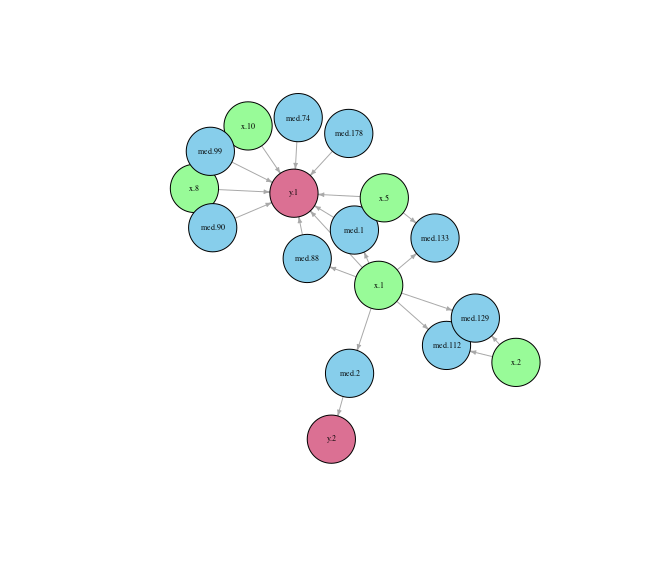## Fit single mvregmed model with user-specified lambda

Below shows how to fit a single model, and what happens with summary when all alpha, beta, and delta are 0.

mvfit <- mvregmed.fit(x, med, y, lambda = 0.4)
summary.mvregmed(mvfit, eps = 0.01)
Warning in summary.mvregmed(mvfit, eps = 0.01): all alpha, beta, delta are zero
NULL

## Plot directed graph of fit model

After edges are created, we use the package igraph to create plots. Because igraph generates graphs based on random seed, it is imporatnt to set a seed to assure the plot can be recreated. We provide the simple function plot.mvregmed.edges to create a basic plot.

## get vertices and edges of graph
mvfit.edges <- mvregmed.edges(mvfit.best, eps = 0.05)

plot.mvregmed.edges(mvfit.edges, x.color = "palegreen", y.color = "palevioletred", med.color = "skyblue",
v.size = 20, seed = 3)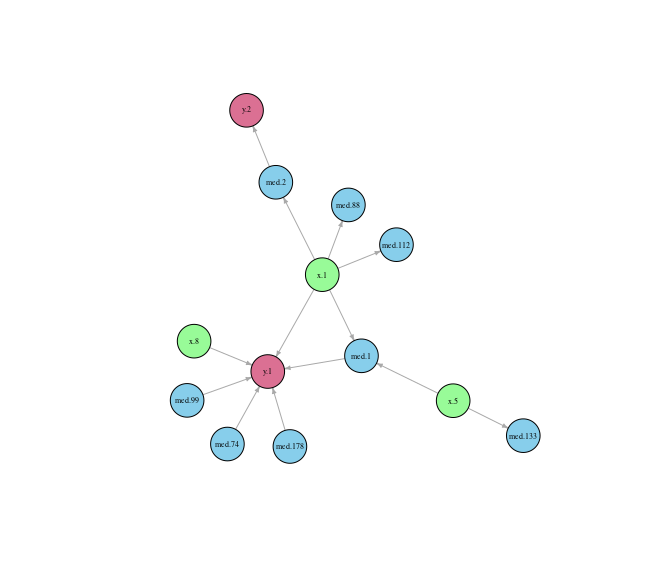To have more control of igraph attributes, we demonstrate below how to use our function mvregmed.graph.attributes to set up initial graph attributes, and then set a variety of igraph attributes in a standard plot function. See igraph for more plot attributes.

gr <- regmed:::mvregmed.graph.attributes(mvfit.edges, x.color = "limegreen", y.color = "palevioletred",
med.color = "royalblue", v.size = 40)
set.seed(3)
plot(gr$gr, vertex.size = gr$vsize, vertex.color = gr\$vcol, vertex.label.font = 2, vertex.label.color = "black",
vertex.label.cex = 0.5, edge.arrow.mode = ">", edge.arrow.size = 0.3)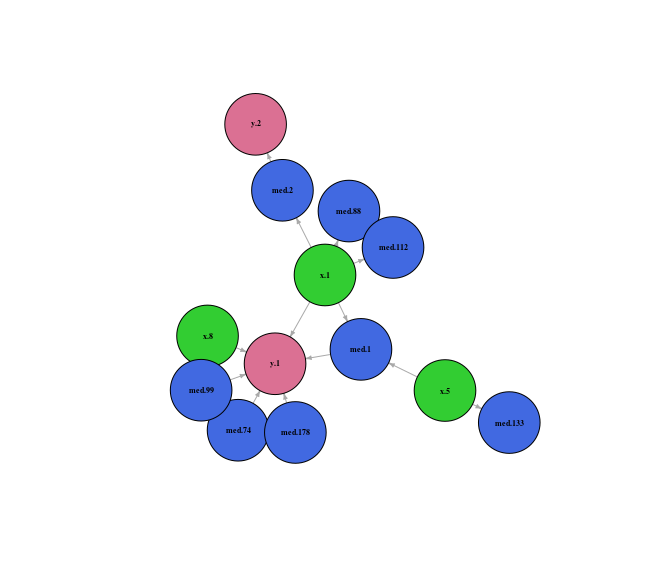## Lavaan sem: Estimate parameters of seleted model without imposing penalty

Like methods for regmed, we provide parallel methods for mvregmed that can be used to setup and fit models with lavaan sem. The 4 key steps are:

• determine edges with mvregmed.edges
• create the model with mvregmed.lavaan.model
• setup the data with mvregmed.lavaan.dat
• fit model with sem
mvmod <- mvregmed.lavaan.model(mvfit.edges, mvfit.best)
mvdat <- mvregmed.lavaan.dat(x, med, y)

mvfit.lavaan <- lavaan:::sem(model = mvmod, data = mvdat)

summary.lavaan(mvfit.lavaan)
       lhs op     rhs    est    se      z pvalue ci.lower ci.upper
1    med.1  ~     x.1  0.462 0.086  5.402  0.000    0.294    0.630
2    med.1  ~     x.5  0.179 0.085  2.104  0.035    0.012    0.346
3    med.2  ~     x.1  0.442 0.075  5.921  0.000    0.295    0.588
4   med.88  ~     x.1 -0.266 0.087 -3.048  0.002   -0.437   -0.095
5  med.112  ~     x.1 -0.315 0.085 -3.699  0.000   -0.482   -0.148
6  med.133  ~     x.5  0.280 0.084  3.345  0.001    0.116    0.444
7      y.1  ~     x.1  0.214 0.103  2.080  0.038    0.012    0.415
8      y.1  ~     x.8  0.126 0.091  1.381  0.167   -0.053    0.305
9      y.1  ~   med.1  0.381 0.093  4.096  0.000    0.199    0.563
10     y.1  ~  med.74 -0.106 0.081 -1.314  0.189   -0.265    0.052
11     y.1  ~  med.99 -0.184 0.077 -2.389  0.017   -0.336   -0.033
12     y.1  ~ med.178 -0.117 0.081 -1.449  0.147   -0.276    0.041
13     y.2  ~   med.2  0.403 0.095  4.242  0.000    0.217    0.589
14     y.1 ~~     y.1  0.532 0.075  7.071  0.000    0.384    0.679
15     y.2 ~~     y.2  0.820 0.116  7.071  0.000    0.593    1.047
16     y.1 ~~     y.2 -0.096 0.067 -1.435  0.151   -0.226    0.035

# Interpretation of lavaan output

The parameters alpha, beta, and delta are determined by regression models indicated by the tilde ($$\sim$$) operator, while variance and covariance parameters are indicated by the double tilde ($$\sim\sim$$) operator. Note that the covariance matrices for x and mediator are assumed fixed based on the values returned from regmed and mvregmed, so only variances and covariances for outcome y are provided. Also note that the z values and p-values from lavaan are marginal effects, not from a joint test of model parameters.